# Python: K Means Cluster

K Means Cluster will be our introduction to Unsupervised Machine Learning. What is Unsupervised Machine Learning exactly? Well, the simplest explanation I can offer is that unlike supervised where our data set contains a result, unsupervised does not.

Think of a simple regression where I have the square footage and selling prices (result) of 100 houses. Taking that data, I can easily create a prediction model that will predict the selling price of a house based off of square footage. – This is supervised machine learning

Now, take a data set containing 100 houses with the following data: square footage, house style, garage/no garage, but no selling price. We can’t create a prediction model since we have no knowledge of prices, but we can group the houses together based on commonalities. These groupings (clusters) can be used to gain knowledge of your data set.

I think seeing it in action will help.

If you want to play along, download the data set here: KMeans1

The data set contains a 1 year repair history of 197 Ultrasound medical devices.

Data dictionary (ID Tag – asset number assigned device, Model – model name of device, WO Count – count of repair work orders, AVG Labor – average labor minutes per repair, Labor Cost – average labor cost per repair, No Problem-  count of repairs where no problem was found, Avg Cost -average cost of parts, Travel – average travel hours per repair, Travel Cost – average travel cost per repair, Department – department that owns the ultrasound device)We want to see what kind of information we can extract from this data.

To do so, we are going to use K Means Clustering.

How does K Means Clustering work? Each row in the table is converted to a vector. Imagine the vectors now graphed in N-dimension space. Next pick the number of clusters you want to create. For each cluster, you will place a  point(a centroid) in space and the vectors are grouped based on their proximity to their nearest centroid.

The calculation to tell proximity is made using geometric means (not arithmetic)- hence the name K-Means Cluster

(each dot below is a row in your table, the colors represent a cluster)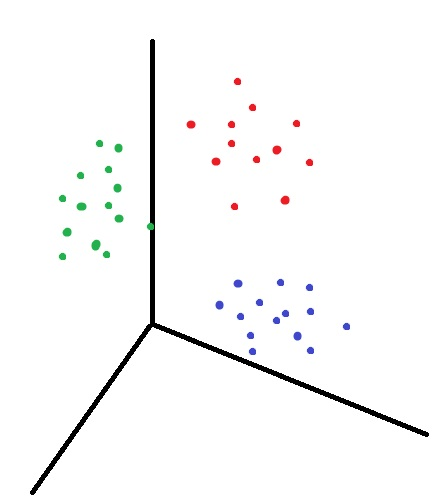## Let’s do it in Python

Import the data.

```import pandas as pd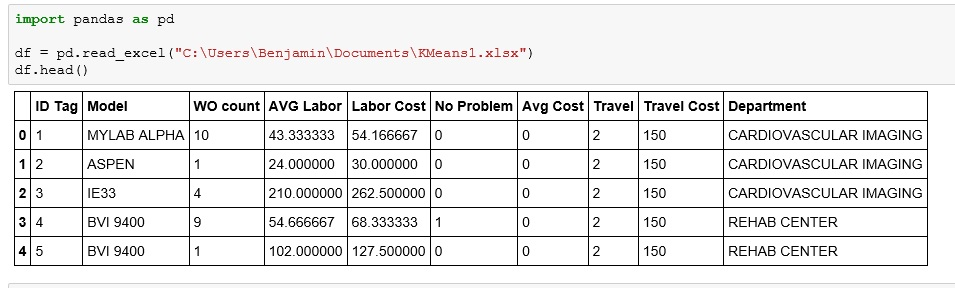Now, we are going to drop a few columns: ID Tag – is a random number, has no value in clustering. Then Model and Department,as they are text and while there are ways to work with the text, it is more complicated so for now, we are just going to drop the columns

```df1 = df.drop(["ID Tag", "Model", "Department"], axis = 1)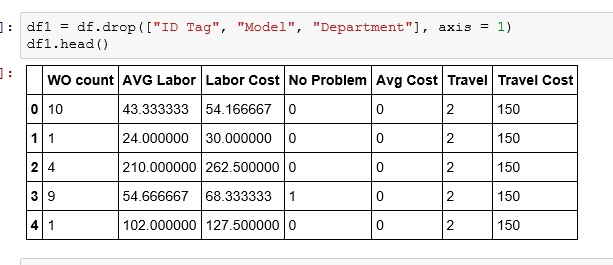Now lets import KMeans from sklearn.cluster

We then initialize KMeans (n_clusters= 4 -no of clusters you want, init=’k-means++’ -sets how the centroids are places. k-means++ is one of the faster methods of centroid placement, n_init=10 – number times the algorithm with run placing new centroids each iteration)

```from sklearn.cluster import KMeans
km = KMeans(n_clusters=4, init='k-means++', n_init=10)```Choosing number of clusters is a bit of an art. Play with it a bit and see how different values play out for you.

Now fit the model

`km.fit(df1)`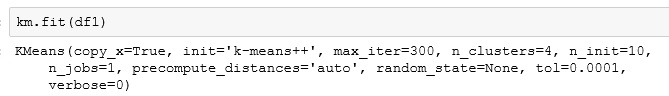Now, export the cluster identifiers to a list. Notice my values are 0 -3. One value for each cluster.

```x = km.fit_predict(df1)
x```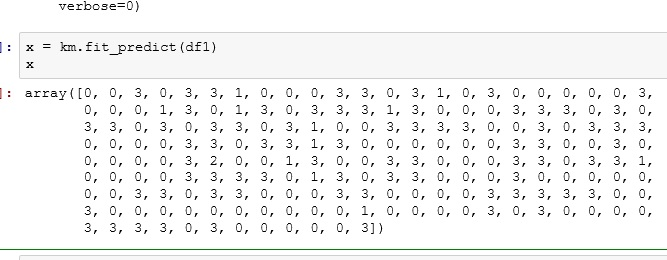Create a new column on the original dataframe called Cluster and place your results (x) in that column

```df["Cluster"]= x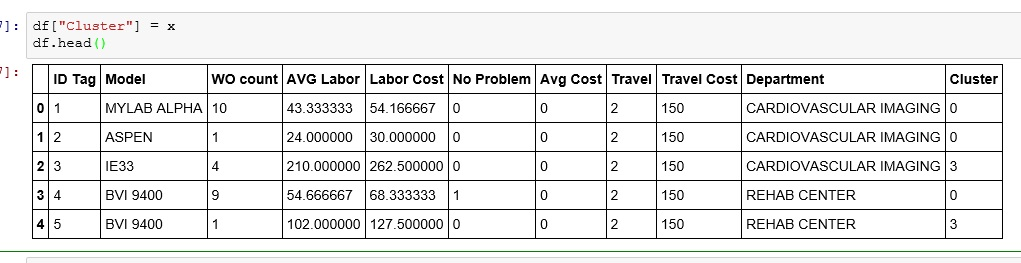```df1 = df.sort(['Cluster'])
df1```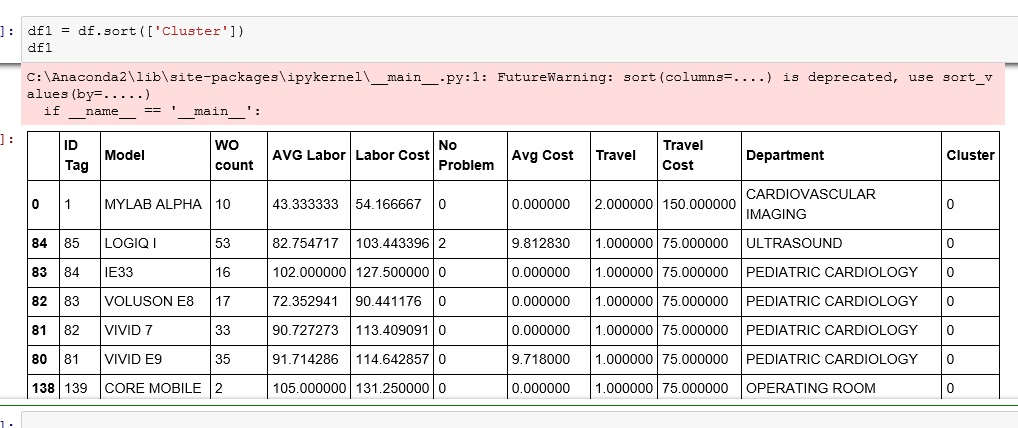Now as you start to examine the data in each cluster, you show start to see patterns emerge.

Below is an example of the patterns I found in the clusters.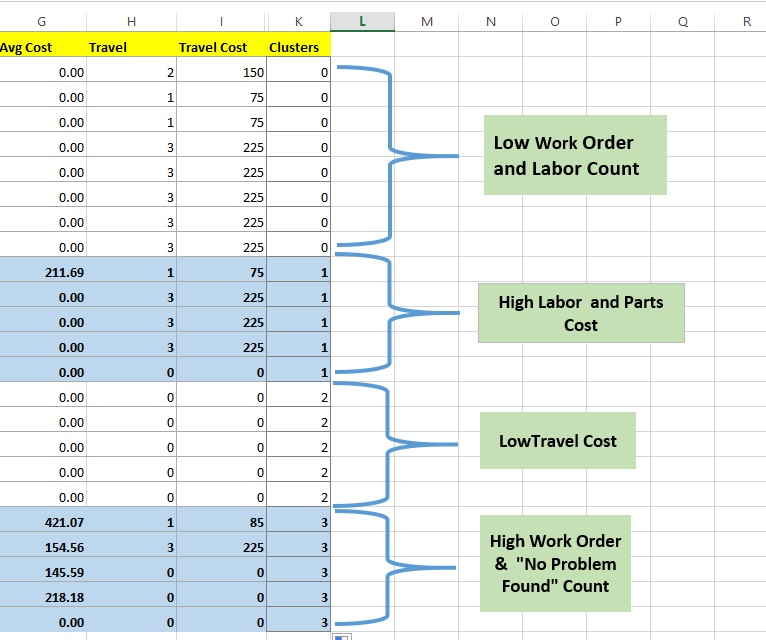Now remember, this is just an INTRODUCTION to unsupervised learning. We will learn more tricks to help you discover the patterns as we move forward.

## 6 thoughts on “Python: K Means Cluster”

1.Jim

It’s the best time to make some plans for the long run and it is time to be happy.
I have read this submit and if I may just I wish
to suggest you few interesting things or advice. Maybe you can write subsequent articles
approximately it!

1.Ben Larson

Thanks Jim, I will work on posting some more articles on unsupervised learning soon.

2.Michael

Very good introduction and easy to understand, thanks!

Kind regards from Germany

3.Arun

Actually I display cluster and centroid points using k-means cluster algorithm.
My problem is animate iteration using k-means algorithm using python not display
If anyone did animate iteration using k-means algorithm in python please send your code to mail_id:arunram860@gmail.com

4.Anonymous

can you please tell me how to name the formed clusters rather than naming them as 0 ,1 ,2 .. etc

5.BestEmil

I see you don’t monetize analytics4all.org, don’t waste your traffic, you
can earn extra bucks every month with new monetization method.
This is the best adsense alternative for any type of